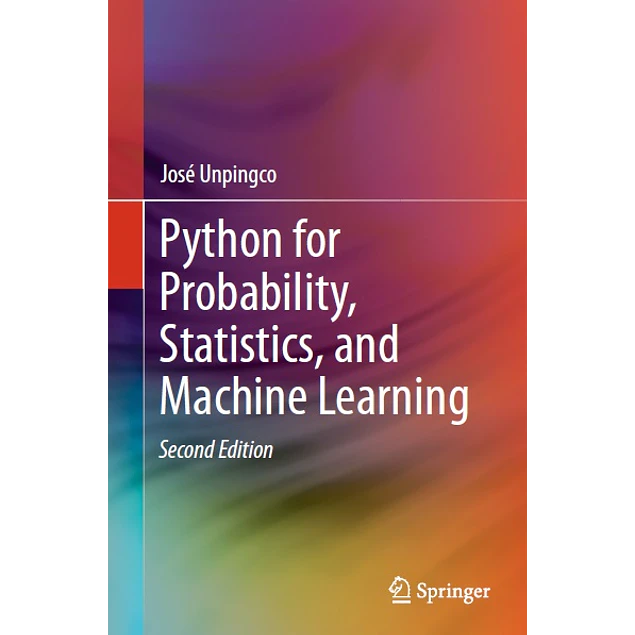## Python for Probability, Statistics, and Machine Learning

20.00\$# Python for Probability, Statistics, and Machine Learning

20.00\$

2nd ed. 2019 Edition

by José Unpingco (Author)

This book, fully updated for Python version 3.6+, covers the key ideas that link probability, statistics, and machine learning illustrated using Python modules in these areas. All the figures and numerical results are reproducible using the Python codes provided. The author develops key intuitions in machine learning by working meaningful examples using multiple analytical methods and Python codes, thereby connecting theoretical concepts to concrete implementations. Detailed proofs for certain important results are also provided. Modern Python modules like Pandas, Sympy, Scikit-learn, Tensorflow, and Keras are applied to simulate and visualize important machine learning concepts like the bias/variance trade-off, cross-validation, and regularization. Many abstract mathematical ideas, such as convergence in probability theory, are developed and illustrated with numerical examples.

This updated edition now includes the Fisher Exact Test and the Mann-Whitney-Wilcoxon Test. A new section on survival analysis has been included as well as substantial development of Generalized Linear Models. The new deep learning section for image processing includes an in-depth discussion of gradient descent methods that underpin all deep learning algorithms. As with the prior edition, there are new and updated *Programming Tips* that the illustrate effective Python modules and methods for scientific programming and machine learning. There are 445 run-able code blocks with corresponding outputs that have been tested for accuracy. Over 158 graphical visualizations (almost all generated using Python) illustrate the concepts that are developed both in code and in mathematics. We also discuss and use key Python modules such as Numpy, Scikit-learn, Sympy, Scipy, Lifelines, CvxPy, Theano, Matplotlib, Pandas, Tensorflow, Statsmodels, and Keras.

This book is suitable for anyone with an undergraduate-level exposure to probability, statistics, or machine learning and with rudimentary knowledge of Python programming.

Year:
2019
Pages:
395
Language:
English
Format:
PDF
Size:
11 MB
Publisher:
Springer
ISBN-10:
3030185443
ISBN-13:
978-3030185442
ASIN:
B07TQCV5VZ
Tag:
Python for Probability, Statistics, and Machine Learning
##### Contact
••••